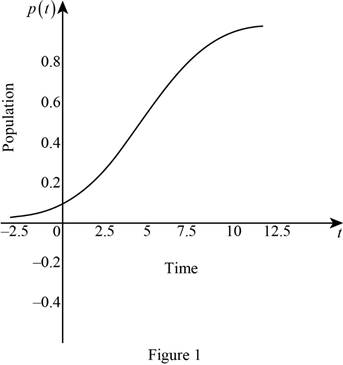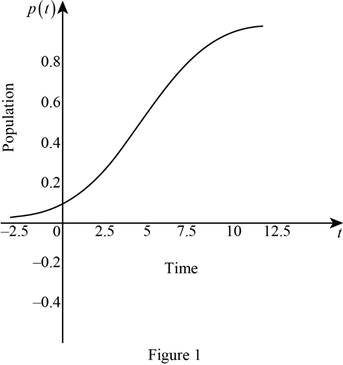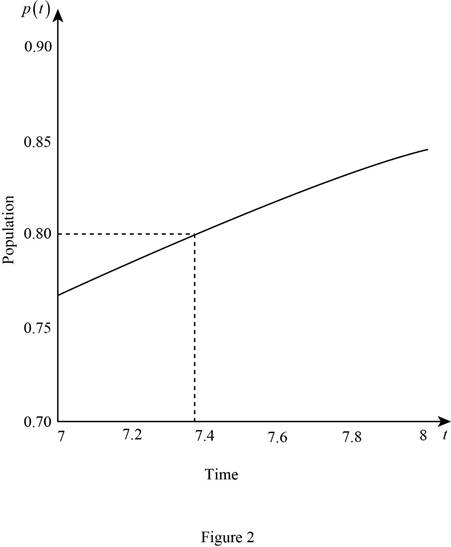# The limit of the function lim t → ∞ p ( t ) .### Single Variable Calculus: Concepts...

4th Edition
James Stewart
Publisher: Cengage Learning
ISBN: 9781337687805### Single Variable Calculus: Concepts...

4th Edition
James Stewart
Publisher: Cengage Learning
ISBN: 9781337687805

#### Solutions

Chapter 3.4, Problem 74E

(a)

To determine

## To find: The limit of the function limt→∞p(t).

Expert Solution

The limit of the function limtp(t)=1.

### Explanation of Solution

Calculation:

Obtain limit of the function.

That is, compute limtp(t).

limtp(t)=limt(11+aekt)=limt(11+aekt)=11+ae=11+a

Simplify further,

limtp(t)=11+0=1

Therefore, the limit of the function limtp(t)=1.

(b)

To determine

### To find: The rate of spread of the rumor.

Expert Solution

The rate of spread of the rumor is akekt(1+aekt)2.

### Explanation of Solution

Given:

The circumstances a rumor spreads according to the equation p(t)=11+aekt.

Derivative rule:

Quotient Rule: ddt(fg)=gddt(f)fddt(g)(g)2

Calculation:

Obtain the derivative of p(t).

p(t)=ddt(p(t))=ddt(11+aekt)

Apply quotient rule (1),

p(t)=(1+aekt)ddt(1)1ddt(1+aekt)(1+aekt)2=(1+aekt)(0)(0+aekt(k))(1+aekt)2=(kaekt)(1+aekt)2=akekt(1+aekt)2

Therefore, the rate of spread of rumor is akekt(1+aekt)2.

(c)

To determine

### To sketch: The graph p(t) and estimate the time such that 80% of the population to hear the rumor.

Expert Solution

Use online graphing calculator and the draw graph of p(t)=11+10e0.5t is shown in the below Figure 1.The rumor spread 80% of the population when t=7.38 seconds.

### Explanation of Solution

Calculation:

Consider p(t)=11+aekt

Substitute a=10 and k=0.5,

p(t)=11+10e0.5t

Use online graphing calculator and the draw graph of p(t)=11+10e0.5t is shown in the below Figure 1.Zooms the graph towards the population p(t)=0.80 as shown below in Figure 2.From Figure 2,it is observed that the rumor spread 80% of the population approximately when t=7.38 seconds.

Obtain time t when p(t)=0.8.

Substitute p(t)=0.8 in p(t)=11+10e0.5t,

0.8=11+10e0.5t1+10e0.5t=10.810e0.5t=1.251e0.5t=0.2510

Take natural logarithm on both sides,

lne0.5t=ln(0.025)0.5t=ln(0.025)t=ln(0.025)0.5t7.3777

Therefore, the rumor spread 80% of the population when t=7.38 seconds.

### Have a homework question?

Subscribe to bartleby learn! Ask subject matter experts 30 homework questions each month. Plus, you’ll have access to millions of step-by-step textbook answers!